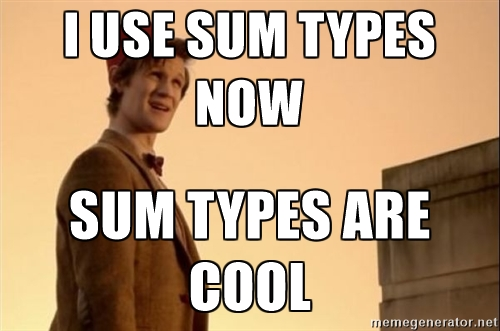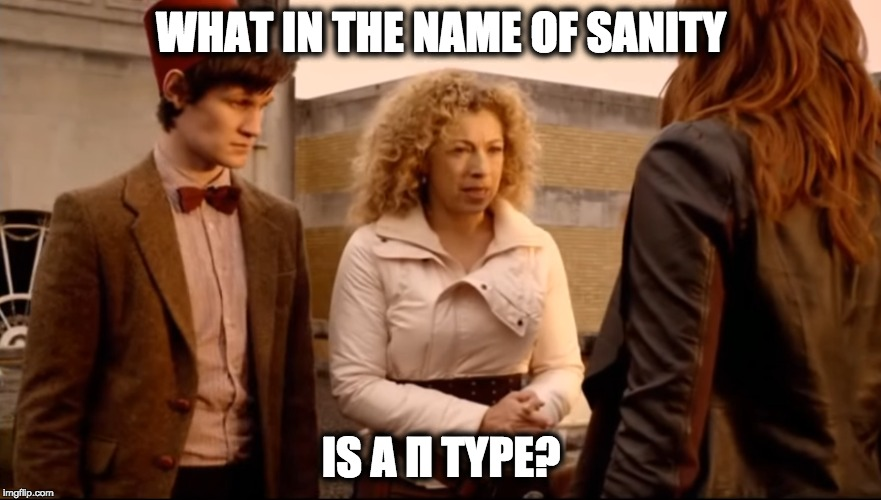# What Are Sum, Product, and Pi Types?

Posted by Manish Goregaokar on March 04, 2017 in programming, rust

You often hear people saying “Language X1 has sum types” or “I wish language X had sum types”2, or “Sum types are cool”.

Much like fezzes and bow ties, sum types are indeed cool.These days, I’ve also seen people asking about “Pi types”, because of this Rust RFC.

But what does “sum type” mean? And why is it called that? And what, in the name of sanity, is a Pi type?

Before I start, I’ll mention that while I will be covering some type theory to explain the names “sum” and “product”, you don’t need to understand these names to use these things! Far too often do people have trouble understanding relatively straightforward concepts in languages because they have confusing names with confusing mathematical backgrounds3.

## So what’s a sum type? (the no-type-theory version)

In it’s essence, a sum type is basically an “or” type. Let’s first look at structs.

struct Foo {
x: bool,
y: String,
}


Foo is a bool AND a String. You need one of each to make one. This is an “and” type, or a “product” type (I’ll explain the name later).

So what would an “or” type be? It would be one where the value can be a bool OR a String. You can achieve this with C++ with a union:

union Foo {
bool x;
string y;
}

foo.x = true; // set it to a bool
foo.y = "blah"; // set it to a string


However, this isn’t exactly right, since the value doesn’t store the information of which variant it is. You could store false and the reader wouldn’t know if you had stored an empty string or a false bool.

There’s a pattern called “tagged union” (or “discriminated union”) in C++ which bridges this gap.

union FooUnion {
bool x;
string y;
}

enum FooTag {
BOOL, STRING
}

struct Foo {
FooUnion data;
FooTag tag;
}

// set it to a bool
foo.data.x = true;
foo.tag = BOOL;

// set it to a string
foo.data.y = "blah";
foo.tag = STRING;


Here, you manually set the tag when setting the value. C++ also has std::variant (or boost::variant) that encapsulates this pattern with a better API.

While I’m calling these “or” types here, the technical term for such types is “sum” types. Other languages have built-in sum types.

Rust has them and calls them “enums”. These are a more generalized version of the enums you see in other languages.

enum Foo {
Str(String),
Bool(bool)
}

let foo = Foo::Bool(true);

// "pattern matching"
match foo {
Str(s) => /* do something with string s */,
Bool(b) => /* do something with bool b */,
}


Swift is similar, and also calls them enums

enum Foo {
case str(String)
case boolean(bool)
}

let foo = Foo.boolean(true);
switch foo {
case .str(let s):
// do something with string s
case .boolean(let b):
// do something with boolean b
}


You can fake these in Go using interfaces, as well. Typescript has built-in unions which can be typechecked without any special effort, but you need to add a tag (like in C++) to pattern match on them.

data Foo = B Bool | S String

-- define a function
doThing :: Foo -> SomeReturnType
doThing (B b) = -- do something with boolean b
doThing (S s) = -- do something with string s

-- call it
doThing (S "blah")
doThing (B True)


One of the very common things that languages with sum types do is express nullability as a sum type;

// an Option is either "something", containing a type, or "nothing"
enum Option<T> {
Some(T),
None
}

let x = Some("hello");
match x {
Some(s) => println!("{}", s),
None => println!("no string for you"),
}


Generally, these languages have “pattern matching”, which is like a switch statement on steroids. It lets you match on and destructure all kinds of things, sum types being one of them. Usually, these are “exhaustive”, which means that you are forced to handle all possible cases. In Rust, if you remove that None branch, the program won’t compile. So you’re forced to deal with the none case, somehow.

In general sum types are a pretty neat and powerful tool. Languages with them built-in tend to make heavy use of them, almost as much as they use structs.

## Why do we call it a sum type?

Here be (type theory) dragons

Let’s step back a bit and figure out what a type is.

It’s really a restriction on the values allowed. It can have things like methods and whatnot dangling off it, but that’s not so important here.

In other words, it’s like4 a set. A boolean is the set $$\{\mathtt{true}, \mathtt{false}\}$$. An 8-bit unsigned integer (u8 in Rust) is the set $$\{0, 1, 2, 3, …. 254, 255\}$$. A string is a set with infinite elements, containing all possible valid strings5.

What’s a struct? A struct with two fields contains every possible combination of elements from the two sets.

struct Foo {
x: bool,
y: u8,
}


The set of possible values of Foo is

$\{(\mathtt{x}, \mathtt{y}): \mathtt{x} \in \mathtt{bool}, \mathtt y \in \mathtt{u8}\}$

(Read as “The set of all $$(\mathtt{x}, \mathtt{y})$$ where $$\tt x$$ is in $$\mathtt{bool}$$ and $$\tt y$$ is in $$\mathtt{u8}$$”)

This is called a Cartesian product, and is often represented as $$\tt Foo = bool \times u8$$. An easy way to view this as a product is to count the possible values: The number of possible values of Foo is the number of possible values of bool (2) times the number of possible values of u8 (256).

A general struct would be a “product” of the types of each field, so something like

struct Bar {
x: bool,
y: u8,
z: bool,
w: String
}


is $$\mathtt{Bar = bool \times u8 \times bool \times String}$$

This is why structs are called “product types”6.

You can probably guess what comes next – Rust/Swift enums are “sum types”, because they are the sum of the two sets.

enum Foo {
Bool(bool),
Integer(u8),
}


is a set of all values which are valid booleans, and all values which are valid integers. This is a sum of sets, $$\tt Foo = bool + u8$$. More accurately, it’s a disjoint union, where if the input sets have overlap, the overlap is “discriminated” out.

An example of this being a disjoint union is:

enum Bar {
Bool1(bool),
Bool2(bool),
Integer(u8).
}


This is not $$\tt Bar = bool + bool + u8$$, because $$\tt bool + bool = bool$$, (regular set addition doesn’t duplicate the overlap).

$\tt Bar = bool + otherbool + u8$

where $$\tt otherbool$$ is also a set $$\tt \{true, false\}$$, except that these elements are different from those in $$\tt bool$$. You can look at it as if

$\tt otherbool = \{true_2, false_2\}$

so that

$\mathtt{bool + otherbool} = \{\mathtt{true, false, true_2, false_2}\}$

For sum types, the number of possible values is the sum of the number of possible values of each of its component types.

So, Rust/Swift enums are “sum types”.

You may often notice the terminology “algebraic datatypes” (ADT) being used, usually that’s just talking about sum and product types together – a language with ADTs will have both.

In fact, you can even have exponential types! The notation A^B in set theory does mean something, it’s the set of all possible mappings from $$B$$ to $$A$$. The number of elements is $${N_A}^{N_B}$$. So basically, the type of a function (which is a mapping) is an “exponential” type. You can also view it as an iterated product type, a function from type B to A is really a struct like this:

// the type
fn my_func(b: B) -> A;

// is conceptually (each possible my_func can be written as an instance of)

struct my_func {
b1: A, // value for first element in B
b2: A, // value for second element in B
b3: A,
// ...
}


given a value of the input b, the function will find the right field of my_func and return the mapping. Since a struct is a product type, this is

$\mathtt{A}^{N_\mathtt{B}} = \tt A \times A \times A \times \dots$

making it an exponential type.

You can even take derivatives of types! (h/t Sam Tobin-Hochstadt for pointing this out to me)

## What, in the name of sanity, is a Pi type?It’s essentially a form of dependent type. A dependent type is when your type can depend on a value. An example of this is integer generics, where you can do things like Array<bool, 5>, or template<unsigned int N, typename T> Array<T, N> ... (in C++).

Note that the type signature contains a type dependent on an integer, being generic over multiple different array lengths.

The name comes from how a constructor for these types would look:

// create an array of booleans from a given integer
// I made up this syntax, this is _not_ from the Rust Pi type RFC
fn make_array(x: u8) -> Array<bool, x> {
// ...
}

// or
// (the proposed rust syntax)
fn make_array<const x: u8>() -> Array<bool, x> {
// ...
}


What’s the type of make_array here? It’s a function which can accept any integer and return a different type in each case. You can view it as a set of functions, where each function corresponds to a different integer input. It’s basically:

struct make_array {
make_array_0: fn() -> Array<bool, 0>,
make_array_1: fn() -> Array<bool, 1>,
make_array_2: fn() -> Array<bool, 2>,
make_array_3: fn() -> Array<bool, 3>,
make_array_4: fn() -> Array<bool, 4>,
make_array_5: fn() -> Array<bool, 5>,
// ...
}


Given an input, the function chooses the right child function here, and calls it.

This is a struct, or a product type! But it’s a product of an infinite number of types7.

We can look at it as

$\texttt{make_array} = \prod\limits_{x = 0}^\infty\left( \texttt{fn()} \mathtt\to \texttt{Array<bool, x>}\right)$

The usage of the $$\Pi$$ symbol to denote an iterative product gives this the name “Pi type”.

In languages with lazy evaluation (like Haskell), there is no difference between having a function that can give you a value, and actually having the value. So, the type of make_array is the type of Array<bool, N> itself in languages with lazy evaluation.

There’s also a notion of a “sigma” type, which is basically

$\sum\limits_{x = 0}^\infty \left(\texttt{fn()} \mathtt\to \texttt{Array<bool, x>}\right)$

With the Pi type, we had “for all N we can construct an array”, with the sigma type we have “there exists some N for which we can construct this array”. As you can expect, this type can be expressed with a possibly-infinite enum, and instances of this type are basically instances of Array<bool, N> for some specific N where the N is only known at runtime. (much like how regular sum types are instances of one amongst multiple types, where the exact type is only known at runtime). Vec<bool> is conceptually similar to the sigma type Array<bool, ?>, as is &[bool].

## Wrapping up

Types are sets, and we can do set-theory things on them to make cooler types.

Let’s try to avoid using confusing terminology, however. If Rust does get “pi types”, let’s just call them “dependent types” or “const generics” :)

Thanks to Zaki, Avi Weinstock, Corey Richardson, and Peter Atashian for reviewing drafts of this post.

1. Rust, Swift, sort of Typescript, and all the functional languages who had it before it was cool.

2. Lookin’ at you, Go.